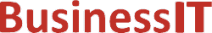O nás     Inzerce     KontaktSpolehlivé informace o IT již od roku 2011[Linux manuál]

# tgamma, tgammaf, tgammal: skutečná funkce gama

Originální popis anglicky: tgamma, tgammaf, tgammal - true gamma function

Návod, kniha: libc math functions

## STRUČNĚ

#include <math.h>

double tgamma(double x);

float tgammaf(float x);

long double tgammal(long double x);

## POPIS / INSTRUKCE

The Gamma function is defined by

Gamma(x) = integral from 0 to infinity of t^(x-1) e^-t dt

It is defined for every real number except for nonpositive integers. For nonnegative integral m one has

Gamma(m+1) = m!

and, more generally, for all x:

Gamma(x+1) = x * Gamma(x)

For x < 0.5 one can use

Gamma(x) * Gamma(1-x) = PI/sin(PI*x)
This function returns the value of the Gamma function for the argument x. It had to be called "true gamma function" since there is already a function gamma() that returns something else.

## CHYBY / ERRORY

In order to check for errors, set errno to zero and call feclearexcept(FE_ALL_EXCEPT) before calling these functions. On return, if errno is non-zero or fetestexcept(FE_INVALID | FE_DIVBYZERO | FE_OVERFLOW | FE_UNDERFLOW) is non-zero, an error has occurred.
A range error occurs if x is too large. A pole error occurs if x is zero. A domain error (or a pole error) occurs if x is a negative integer.

C99.

## SOUVISEJÍCÍ

gamma(3), lgamma(3)
 2002-08-10 GNU
©2011-2020 BusinessIT.cz, ISSN 1805-0522 | Názvy použité v textech mohou být ochrannými známkami příslušných vlastníků.
Provozovatel: Bispiral, s.r.o., kontakt: BusinessIT(at)Bispiral.com | Inzerce: Best Online Media, s.r.o., zuzana@online-media.cz
O vydavateli | Pravidla webu BusinessIT.cz a ochrana soukromí | pg(8445)